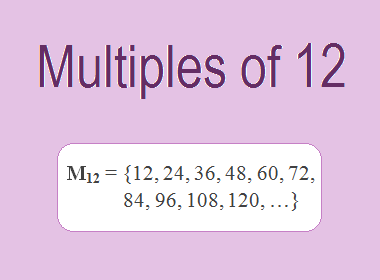# Multiples of 12The Multiples of 12 are 12, 24, 36, 48, 60, 72, 84, 96, 108, 120, … i.e. M12 = {12, 24, 36, 48, 60, 72, 84, 96, 108, 120, …}. Multiples of 12 are the series of numbers obtained by multiplying 12 with the natural numbers.

12 × 1 = 12

12 × 2 = 24

12 × 3 = 36

12 × 4 = 48

12 × 5 = 60

12 × 6 = 72

12 × 7 = 84

12 × 8 = 96

12 × 9 = 108

12 × 10 = 120

And so on.

From this series, we observe that the multiples of 12 can be derived by multiplying 12 by natural numbers in ascending order. These multiples form an arithmetic sequence with a common difference of 12.

## Properties and Patterns of Multiples of 12

Multiples of 12 possess several interesting properties and patterns that make them unique. Here are a few notable ones:

1.    Divisibility by 12: One remarkable property of multiples of 12 is that they are divisible by 12 without leaving any remainder. This property is due to the fact that 12 is a divisor of all its multiples. For example, 48 is a multiple of 12 since it can be divided by 12 to yield 4 as the quotient.

2.    Even Numbers: All multiples of 12 are even numbers. This is because 12 itself is an even number (divisible by 2), and when we multiply an even number by another integer, the result will always be an even number.

3.    Common Factors: Multiples of 12 share common factors with 12 itself. The factors of 12 include 1, 2, 3, 4, 6, and 12. Therefore, any multiple of 12 will also be divisible by these factors. This property is useful when simplifying fractions or finding common denominators.

4.    Repeating Units Digit: One interesting pattern observed in multiples of 12 is that their units digit repeats in a cyclic manner. Starting with 12 itself, the units digit follows a pattern of 2, 4, 6, 8, and then repeats. For example, 12, 24, 36, 48, 60, and so on. This pattern arises due to the cyclical nature of the units digit in multiplication.

5.    Doubling Effect: Another notable pattern is the doubling effect. Each successive multiple of 12 is twice the preceding multiple. For instance, 12 is twice 6, 24 is twice 12, and so on. This doubling effect occurs because multiplying by 12 is equivalent to multiplying by 2 and then multiplying by 6.

********************

********************

## Applications of Multiples of 12

The study of multiples of 12 finds applications in various fields, including mathematics, science, and everyday life:

1.    LCM: One of the applications of multiples of 12 is in finding the lowest common multiple (LCM) of two or more numbers. The LCM is the lowest multiple that two or more numbers have in common. For example, to find the LCM of 12 and 15, we need to find the multiples of both numbers and identify the lowest multiple they have in common. The multiples of 12 are 12, 24, 36, 48, 60, 72, 84, 96, 108, 120 … etc. The multiples of 15 are 15, 30, 45, 60, 75, 90, 105, 120, 135, 150, … etc. The lowest multiple that they have in common is 60. Therefore, the LCM of 12 and 15 is 60.

2.    Time Measurement: The concept of 12-based divisions is ingrained in our daily lives through the 12-hour clock system. It consists of two sets of 12 hours each, making a total of 24 hours in a day. This system is widely used worldwide, aiding in the organization and synchronization of various activities.

3.    Measurement Conversions: Multiples of 12 are frequently utilized for conversion purposes. For example, 12 inches make up one foot, and 12 feet make up one yard. The use of these multiples simplifies measurement conversions, making them more manageable and efficient.

4.    Calendar Systems: Many calendars, such as the Gregorian calendar, follow a structure with 12 months in a year. This division allows for the efficient organization and tracking of time, providing a common framework for scheduling events and planning activities.

## Conclusion:

The Multiples of 12 are the numbers obtained by multiplying 12 with natural numbers. The multiples of 12 are 12, 24, 36, 48, 60, 72, 84, 96, 108, 120 … etc. The multiples of 12 have various properties, such as divisibility by 12, even numbers, common factors, repeating units digit, doubling effect, etc. The multiples of 12 have several applications in mathematics, such as finding the LCM, time measurement, measurement conversions, and calendar systems.